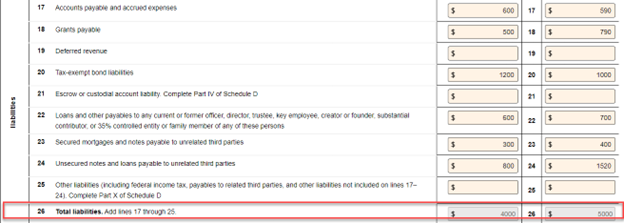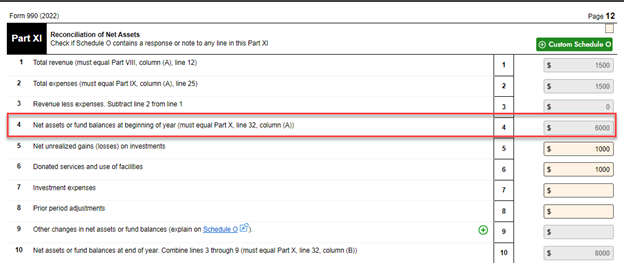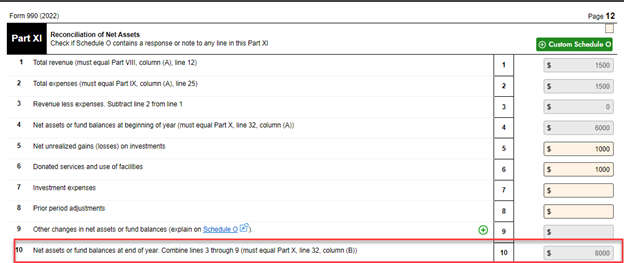# How can I complete assets, liabilities, and, net assets and fund balance on 990 forms? (Use Case) 524 views | Last modified 5/13/2023 1:17:08 PM EST |

Applicable To
990-PF 990-N 990-EZ 990 8868 1120-POL

The purpose of a Balance Sheet on Form 990 is to provide the IRS with a summary of your organization’s financial status. The Balance Sheet comprises 3 sections,

1. Assets (Section 1)  - The resources owned by your Organization that have a financial value.
2. Liabilities (Section 2) - Debt or financial obligations of your Organization
3. Net Assets or Fund Balances (Section 3) - The assets left after deducting the liabilities.

You should report the value of these financial elements at the beginning (Column A) and end of the tax year (Column B).

The total assets you reported in section 1 must match the sum of total liabilities and net assets or fund balances reported in sections 2 and 3, respectively.

Which means

 Total Assets (Line 16) = Total liabilities (Line 26) and net assets/fund balances (Line 32)

For Example, the Organization ABC Charities has the asset values as shown below.The Total assets of the Organization (Line 16)

• Beginning of the tax year (A) = \$10,000
• Year-end (B) = \$13,000

Now, here are the liabilities that the Organization had during the corresponding tax year.The Total Liabilities of the Organization (Line 26)

• Beginning of the tax year (A) = \$4,000
• Year-end (B) = \$5,000

This Organization doesn’t follow the accounting standard FASB ASC 958, and therefore they have reported their net assets on Lines 29, 30, and 31.The Total Net assets or Fund Balances (Line 32)

• Beginning of the tax year (A) = \$6,000
• Year-end (B) = \$8,000

Now, in the last line of the section (Line 33), you can add lines 26 (Total Liabilities) and 32 (Total Net Assets or Fund Balances) to derive Total liabilities and Net assets/fund balances.

Total liabilities and Net assets/fund balances (Line 33) = Line 26 + Line 32

Beginning of the tax year (A)  = \$4,000 + \$6,000 = \$10,000

End of the tax year (B) = \$5,000 + \$8,000 = \$13,000

Now, you can see that the values on Line 16 (A and B) are equal to Line 33 (A and B).Therefore,

 Line 16 (Total Assets) = Line 33 (Total liabilities and Net assets/fund balances)

Now, we can figure out how to balance the value of net assets in the Reconciliation of Net Assets (Part XI).

In this part, the values of total revenues and expenses will be automatically fetched from the previous parts.

Then, you are required to mention the Total Net Assets or fund balances value of your Organization at the beginning of the tax year. (This value should be equal to the Total Net Assets or fund balances value reported last year).

Also, this value will be pre-fetched from Part X, Line 32, A (Total Net Assets of Fund Balances at the beginning of the tax year).

 Part XI, Line 4 (Total net assets or fund balances at the year-beginning)  = Part X, Line 32 - A (Total net assets or fund balances at the year-beginning)Now, you can report the values of net unrealized gains (or losses), donated services, investment expenses, adjustments, and other changes in net assets. Based on that, the Total Net Assets or Fund Balances value at the end of the tax year will be calculated in Line 10.

Earlier, we mentioned the Total Net Assets or Fund Balance at the end of the year (Line 32, B) as \$8,000.  This means the value obtained on Line 10 must also be the same.

 Part XI, Line 10 (Total net assets or fund balances at the year-end)  = Part X, Line 32- B (Total net assets or fund balances at the year-end)Here, you can see both the values are equal. So, that’s it, we are done with the balance sheet and reconciliation.